Algebraic General Topology. Vol 1: Paperback / E-book || Axiomatic Theory of Formulas: Paperback / E-book

As an Amazon Associate I earn from qualifying purchases.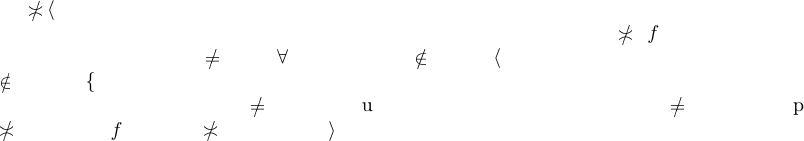Identity St aroids
by Victor Porton
Email: porton@narod.ru
Web: http://www.mathematics21.org
1 Draft status
This text is a draft.
Read my book  before reading this. Well, most p robably I will integrate materials from this
article into my book.
Proposition 1.
hf i
k
X | X up
Q
in \{k }
A
i
;
Q
in \{k }
Z
i
X
is a ﬁlter base on A
k
for every famil y
(A
i
; Z
i
) of ﬁltrators where i n for s ome index set n (provided that f is a multifuncoid of the form
A and k n and every Z
i
for i n \ {k} is a ﬁlter base and X
Q
in \{k}
A
i
).
Proof. Let K, L {hf i
k
X | X up X }. Then there exist X , Y up X such that K = hf i
k
X,
L = hf i
k
Y . We can take Z up X such that Z X , Y . Then evidently hf i
k
Z K and hf i
k
Z L
and hf i
k
Z {hf i
k
X | X up X }.
Proposition 2. h f i
k
X =
d
X up X
hf i
k
X for a ﬁltrator
Q
in \{k}
F
i
;
Q
in \{k}
P
i
(i n
for some index set n) where every Z
i
is a boolean lattice, k n, and X
Q
in \{k}
F
i
.
Proof. F
k
is separable by obvious 4.136??. (F
k
; P
k
) is with separable core by theorem 4.112??.
Y
h f i
i
X X {(i; Y) } GR [ f]⇔X {(i; Y)} GR [f]up(X {(i; Y)}) GR [f]
X up X , Y up Y: X {(i; Y )} GR [f]⇔∀X up X , Y up Y: Y
hf i
i
X X up X ,
Y up Y: Y hf i
i
X
0 Y up Y: 0
{Y hf i
i
X | X up X } Y up Y:
0
hY i{hf i
i
X | X up X } (by properties of generalized ﬁlter bases)⇔∀Y up Y:
d
hY i{hf i
i
X | X up X }
0 Y up Y: Y
d
{hf i
i
X | X up X }
0 Y up Y:
Y
d
X up X
hf i
i
X Y
d
X up X
hf i
i
X; so h f i
i
X =
d
X up X
hf i
i
X.
3 On pseudofuncoids
Deﬁnition 3. Pseudofuncoid from a set A to a set B is a relatio n f between ﬁlters on A and B
such that:
¬(I f 0), I J f K I f K J f K (for every I , J F(A), K F(B)),
¬(0 f I), K f I J K f I K f J (for every I , J F(B), K F(A)).
Obvious 4. Pseudofuncoid is just a staroid of the form (F(A); F(B)).
Obvious 5. [f] is a pseudofuncoid for every funcoid f.
Example 6. If A and B are inﬁnite sets, then t he re exist two diﬀerent pseudofuncoids f and g
from A to B such that f (P × P) = g (P × P) = [c](P × P) for some funcoid c.
Remark 7. Considering a pseudofuncoid f as a staroid, we get f (P × P) = f.
1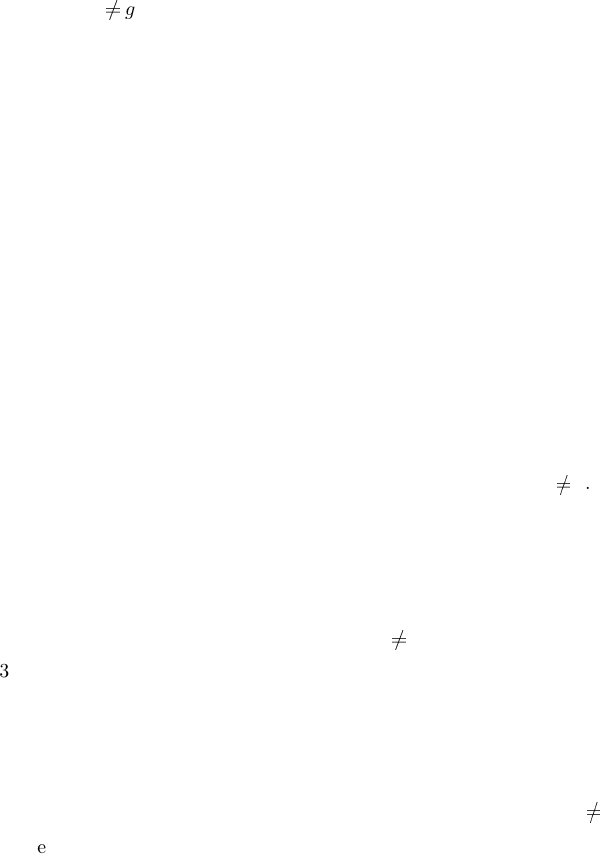Proof. Take
f =
(X ; Y) | X F(A), Y F(B),
\
X and
\
Y are inﬁnite
and
g = f {(X ; Y) | X F(A), Y F(B), X a, Y b}
where a and b are nontrivial ultraﬁlters on A and B correspo ndingly, c is the funcoid deﬁned by
the relation
[c]
= δ = {(X; Y ) | X PA, Y PB , X and Y are inﬁnite}.
First prove that f is a pseudofuncoid. The formulas ¬(I f 0) and ¬(0 f I) are obvious. We have
I J f K
T
(I J ) and
T
Y are inﬁnite
T
I
T
J and
T
Y are inﬁnite(
T
I or
T
J is
inﬁnite)
T
Y is inﬁnite(
T
I and
T
Y are inﬁnite) (
T
J and
T
Y are inﬁnite) I f K J f K.
Similarly K f I J K f I K f J . So f is a pseudofuncoid.
Let now prove that g is a pseudofuncoid. The formulas ¬(I g 0) and ¬(0 g I) are obvious. Let
I J g K. Then either I J f K and then I J g K or I J a and then I a J a thus
having I g K J g K. So I J g K I g K J g K. The reverse implication is obvious. We have
I J g K I g K J g K and similarly K g I J K g I K g J . So g is a pseudofuncoid.
Obviously f
g (a g b but not a f b).
It remains to prove f (P × P) = g (P × P) = [c](P × P). Really, f (P × P) = [c](P × P)
is obvious. If (
A
X;
B
Y ) g (P × P) then either (
A
X;
B
Y ) f (P × P) or X up a, Y up b,
so X and Y are inﬁnite and thus (
A
X;
B
Y ) f (P × P). So g (P × P) = f (P × P).
Remark 8. The above c ounter-example shows that pseudofuncoids (and more generally, any
staroids on ﬁlters) are “second class” objects, they are not full-ﬂedged because they don’t bijectively
correspond to funcoids and the ele gant funcoids theory does not apply to them.
From the above it follows that staroids on ﬁlters do not correspond (by restriction) to staroids
on principal ﬁlters (or staroids on se ts).
4 Complete staroids and multifuncoids
4.1 Complete free stars
Deﬁnition 9. Let A be a poset. Complete free sta rs on A are s uch S P A that the least element
(if it e xists) is not in S and for every T PA
Z A: (X T : Z X Z S) T S
.
Obvious 10. Every complete free star is a free star.
Proposition 11. S PA where A is a poset is a complete free star iﬀ all the following:
1. The least element (if it exists) is not in S.
2. Z A: (X T : Z X Z S) T S
.
3. S is an upper set.
Proof.
. (1) and (2) are obvious. S is an upper s et because S is a free star.
. We need to prove that
Z A: (X T : Z X Z S) T S
.
Let X
T S. Then X T : Z X Z X
Z S because S is an upper set.
Proposition 12. Let S be a complete lattice. S P A is a complete fre e star iﬀ all the following:
1. The least element (if it exists) is not in S.
2 Section 4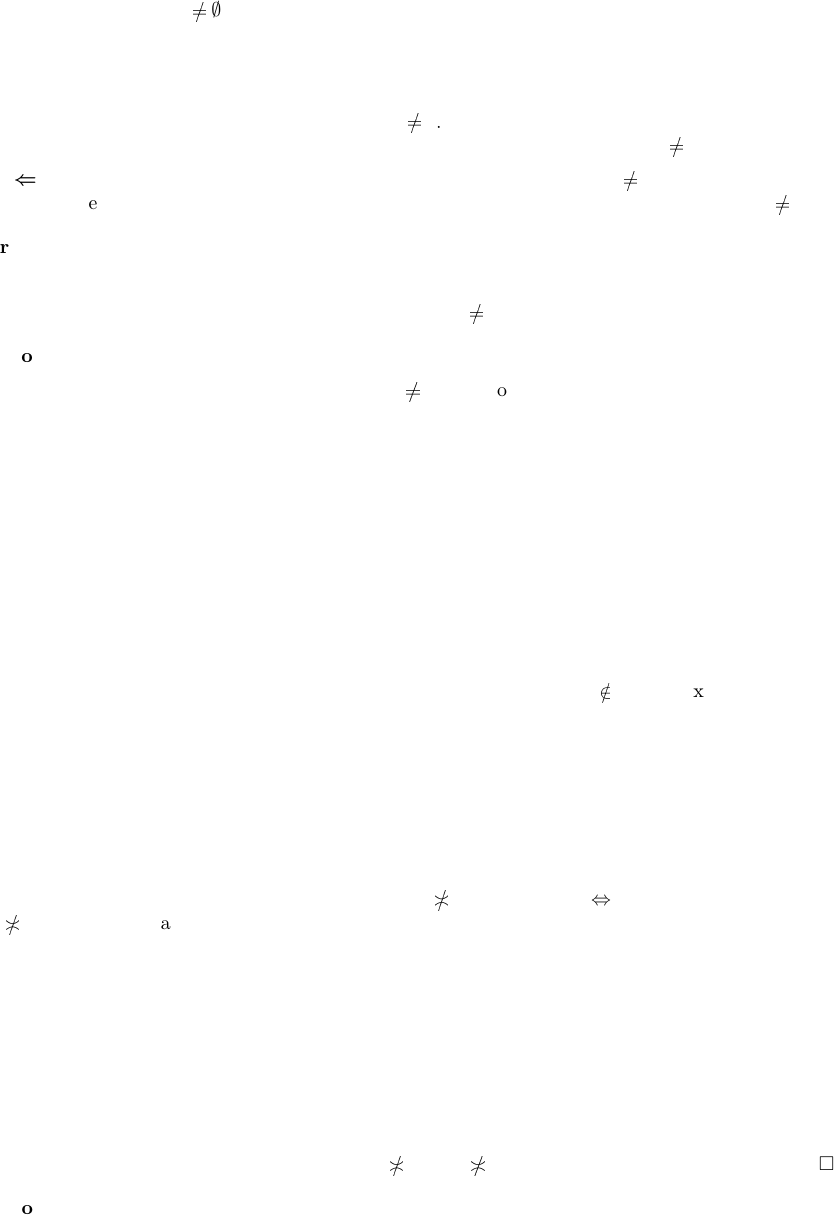2.
F
T S T S
for e very T PS.
3. S is an upper set.
Proof.
. We need to prove only
F
T S T S
. Let
F
T S. Because S is an upper set, we
have X T : Z X Z
F
T Z S from which we conclude T S
.
. We need to prove only Z A: ( X T : Z X Z S) T S
.
Really, if Z A: (X T : Z X Z S) then
F
T S and thus
F
T S T S
.
Proposition 13. Let A be a complete lattice. S PA is a complete free star iﬀ the least element
(if it e xists) is not in S and for every T PA
G
T S T S
.
Proof.
. We need to prove only
F
T S T S
what follows from that S is an upper set.
. We need to prove only that S is an upper set. To prove this we can use the fact that S is
a free star.
4.1.1 Completely s tarrish posets
Deﬁnition 14. I will call a poset completely starrish when the full star a is a free star for every
element a of this poset.
Obvious 15. Every completely starrish poset is starrish.
Proposition 16. Every co mplete join inﬁnite distributive lattice is starrish.
Proof. Let A be a join inﬁnite dis tributive lattice, a A. Obviously 0
⋆a (if 0 exists); obviously
a is an upper set. If
F
T a, then (
F
T ) a is non-least that is
F
ha i T is no n-least what is
equivalent to a x being non-least for some x T that is x a.
Theorem 17. If A is a comple tely starrish complete lattice lattice then
atoms
G
T =
[
hatomsiT .
for every T PA.
Proof. For every ato m c we have: c atoms
F
T c
F
T
F
T c X T : X c X T :
X
c X T : c atoms X c
S
hatomsiT .
4.2 More on free stars and complete free stars
Obvious 18. F = F for an element F of down-aligned ﬁnitely meet closed ltrator.
Corollary 19. F = F for every ﬁlter F on a poset.
Proposition 20. F = F for an e lement F of a ﬁltrator with separable core.
Proof. X F up X F X X : X
F X
F X F.
Corollary 21. F = F for every ﬁlter F on a distributive lattice with least element.
Proposition 22. For a semiﬁltered, star-separable, down-aligned ﬁltrator (A; Z) with ﬁnitely meet
closed and separable core where Z is a complete boolean lattice and both Z and A are atomistic
lattices the following conditions are equivalent for any F A:
1. F Z.
Complete staroids and multifuncoids 3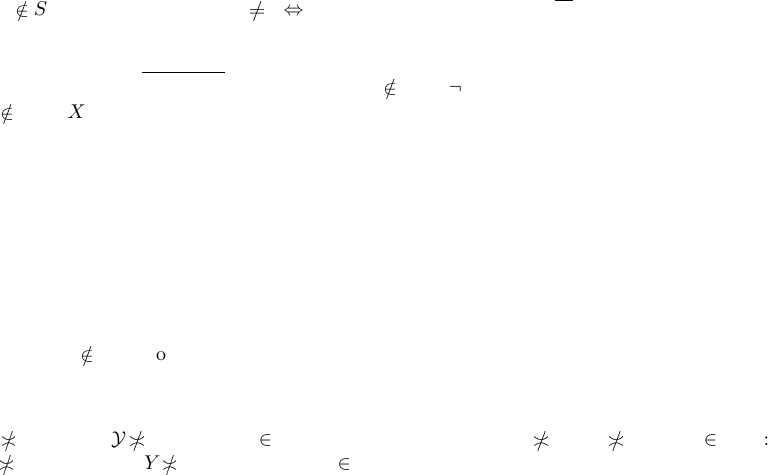2. F is a complete free star on Z.
3. F is a complete free star on F.
Proof.
(1)(2). That F does not contain the least element is obvious. That F is a n upper set is
obvious. So it remains to apply theorem 4.53??.
(2)(3). That F does not contain the least element is obvious. That F is an upper set is
obvious. So it remains to apply theorem 4.53??.
(3)(1). Apply theorem 4.53??.
Corollary 23. For a ﬁlter F F on a complete atomic boolean lattice the following conditions
are equivalent:
1. F P.
2. F is a complete free star on P.
3. F is a complete free star on F.
Theorem 24. Let Z be a boolean lattice. For any set S P P there exists a principal ﬁlter A
such that A = S i S is a complete free star (on P).
Proof.
. From the previous theorem.
. 0
P
S and
F
T S T S
X T : X S. Take A = {X | X P \ S }. We will
prove that A is a prin c ipal ﬁlter. That A is a ﬁlter foll ows from properties of free stars. It
remains to show that A is a principa l ﬁlter. It follows from the following equivalence:
d
P
A A
F
P
h¬iA
A
F
P
h¬iA
S ¬∃X h¬iA: X S X h¬iA:
X
S X A: X A 1.
Proposition 25.
1. If S is a free star on A then S is a free star on Z, p rovided that Z is a join-s e milattice and
the ﬁltrator (A; Z) is down-aligned and with ﬁnitely join-closed core.
2. If S is a free star on P then S is a fr e e star on F, provided that Z is a boolean lattice.
Proof.
1. X
Z
Y S X
Z
Y S X
A
Y S X S Y S X S Y S for every
X , Y Z; 0
S is obvious.
2. There exists a ﬁlter F such that S = F. For every ﬁlters X , Y F
X
A
Y S up(X
A
Y) S K up(X
F
Y): K F K up(X
F
Y):
K
F X
F
Y
F X
F
Y F X F Y F X
F Y
F X up X :
X
F Y up Y:Y
F X upX : X F Y up Y:Y F up X S up Y S
X S Y S;
0 S up 0 S 0 S what is false.
Corollary 26. If S is a free star on F then S is a free sta r on P, provided that P is a join-
semilattice.
Proposition 27.
1. If S is a complete free star on A then S is a complete free star on Z, provided that Z is
a complete lattice and the ﬁltrat or (A; Z) is down-aligned and with join-closed core.
2. If S is a complete free star on P then S is a complete free sta r on F, provided that Z is
a boolean lattice.
4 Section 4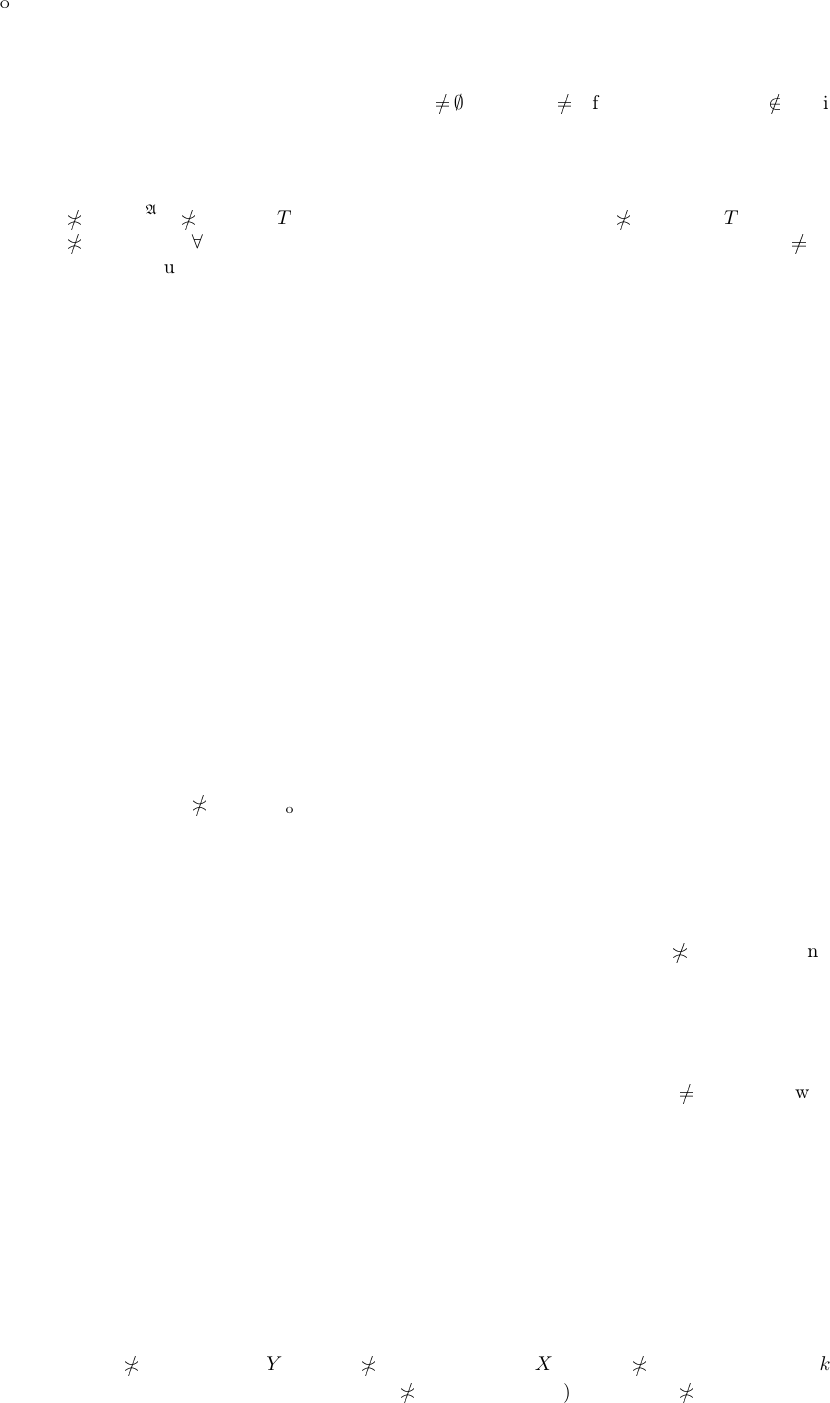Proof.
1.
F
Z
T S
F
Z
T S
F
A
T S T S
T S
for every T P Z; 0
S is
obvious.
2. There exists a principal ﬁlter F such that S = F.
F
A
T S up
F
A
T S K up
F
A
T : K F K up
F
A
T :
K
F
F
A
T
F
F
A
T F ∃K T : K F ∃K T : K
F ∃K T K up K:
K
F ∃K T K up K: K F ∃K T : up K S ∃K T : K S T S
.
0 S up 0 S 0 S what is false.
Corollary 28. If S is a complete free star on F then S is a complete free star on P, provided
that Z is a complete lattice.
5 Complete staroids and multifuncoids
Deﬁnition 29. Consider an indexed family (A
i
; Z
i
) of ﬁltrators. A pre-staroid f of the f orm
Q
Z is complete in argument k arity f when (val f )
k
L is a comple te free star for every
L
Q
i(arity f )\{k}
Z
i
.
Deﬁnition 30. Consider an indexed family (A
i
; Z
i
) of ﬁltrators and pre-multifuncoid f is of
the form
Q
A. Then f is complete in argument k arity f iﬀ hf i
k
L Z
k
for every family
L
Q
i(arity f )\{k}
Z
i
.
Proposition 31. Consider an indexed family (F
i
; Z
i
) o f primary ﬁltrators over boolean lattices.
Let f be a pre-multifuncoid of the form A and k arity f. The following are equivalent:
1. Pre-multifuncoid f is complete in argument k.
2. Pre-staroid [f ] is complete in argument k.
Proof. L GR [f ]L
i
hf i
i
L|
(dom L)\{i}
;
(val [f ])
k
L = hf i
k
L by the theorem ??17.81.
So (val [f ])
k
L is a complete free star iﬀ hf i
k
L Z
k
(proposition 22) for every L
Q
i(arity f )\{k}
Z
i
.
Example 32. Consider funcoid f = id
FCD(U)
. It is obviously complete in ea ch its two a rguments.
Then [f] is not complete in each of its two arguments because (X ; Y) [f]⇔X
Y what does not
generate a complete free star if one of the argu ments (say X ) is a ﬁxed nonprincipa l ﬁlter.
Theorem 33. Consider a semiﬁltered, st ar-separable, down-aligned ﬁltrator (A; Z) with ﬁnitely
meet clos e d and separable core where Z is a complete boolean lattice and both Z a nd A are atomistic
lattices.
Let f be a multifuncoid of the aforementioned form. Let k, l arity f and k
l. The following
are equivalent:
1. f is c omplete in t he argument k.
2. hf i
l
(L {(k;
F
X)}) =
F
xX
hf i
l
(L {(k; x)}) for every X PZ
k
, L
Q
i(arity f )\{k,l}
Z
i
.
3. hf i
l
(L {(k;
F
X)}) =
F
xX
hf i
l
(L {(k;x)} ) for every X P A
k
, L
Q
i(arity f)\{k,l}
Z
i
.
Proof.
(3)(2). Obvious.
(2)(1). Let Y Z.
F
X
hf i
k
(L {(l; Y )}) Y
hf i
l
(L {(k;
F
X)}) Y
F
xX
hf i
l
(L {(k;
x)}) (proposition 4.144??)⇔∃x X: Y
hf i
l
(L {(k; x)}) x X: x
hf i
k
(L (l; Y )).
Complete staroids and multifuncoids 5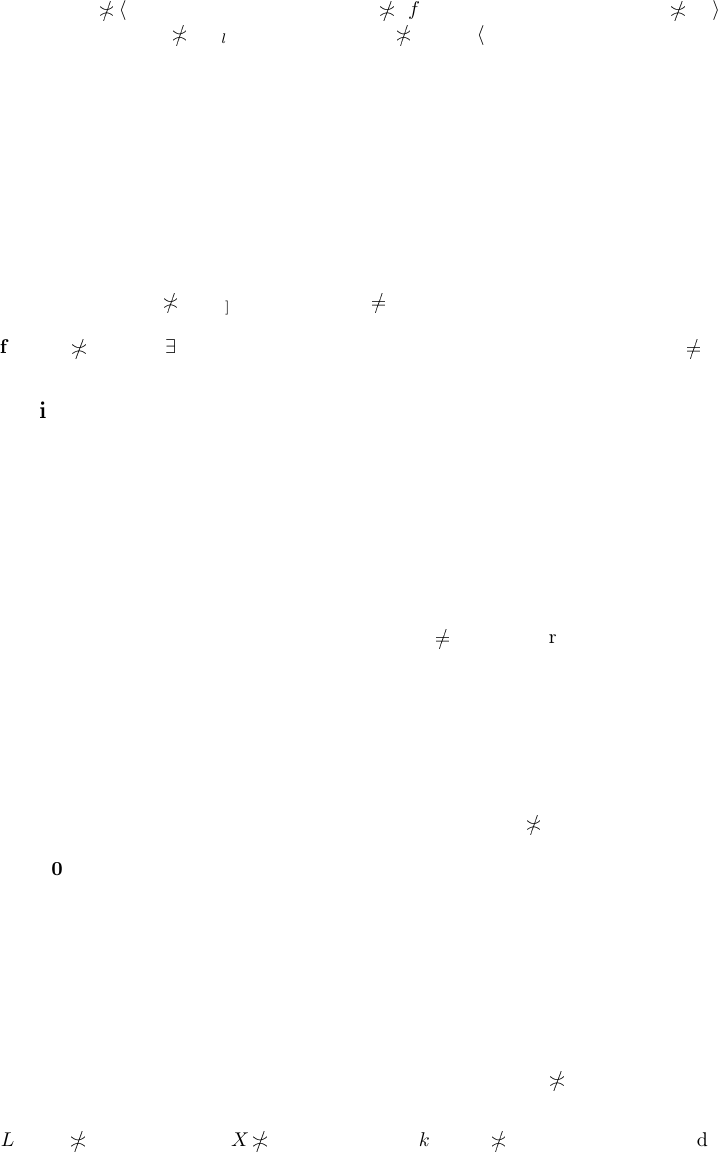It is equivalent (proposition 22 and the fact that [f ] is an upper se t) to hf i
k
(L {(l ; Y )})
being a principal ﬁlter and thus (val [f])
l
L being a complete free star.
(1)(3). Y
hf i
l
(L {(k;
F
X)})
F
X
hf i
k
(L {(l; Y )}) x X: x
hf i
k
(L {(l;
Y )}) x X: Y
hf i
l
(L {(k; x)}) Y
F
xX
hf i
l
(L {(k; x)}) for every principal
Y .
6 Identity staroids and multifuncoids
6.1 Identity relations
Denote id
A[n]
= {(λi n: x) | x A} = {n × {x} | x A} the n-ary identity relation on a set A (for
each index set n).
Proposition 34.
Q
X
id
A[n]
T
in
X
i
A
.
Proof.
Q
X
id
A[n]
t A: n × {t}
Q
X t Ai n: t X
i
T
in
X
i
A
.
6.2 Universal deﬁnitions of identity st aroids
Consider a ﬁltrator (A; Z) and A A.
I will deﬁne below small identity staroids id
A[n]
Strd
and big identity staroids ID
A[n]
Strd
. That they are
really staroids and even completary staroids (under certain conditions) is proved below.
Deﬁnition 3 5. Consider a ﬁltrator (A; Z). Let Z be a complete lattice. Let A A, let n be an
index set.
form id
A[n]
Strd
= Z
n
; L GR id
A[n]
Strd
d
in
Z
L
i
A .
Obvious 36. X GR id
A[n]
Strd
A up A:
d
in
Z
X
i
A
0 if our ﬁltrator is with separable core.
Deﬁnition 37. The subset X of a poset A has a nontrivial lower bound (I denote this predicate
as MEET(X)) iﬀ there is nonleast a A such that x X: a x.
Deﬁnition 38. Staroid ID
A[n]
Strd
(for any A A whe re A is a poset) is deﬁned by the formulas:
form ID
A[n]
Strd
= A
n
; L GR ID
A[n]
Strd
MEET({L
i
| i n} {A} ).
Obvious 39. If A is complete latt ic e , then L GR ID
A[n]
Strd
d
L
A.
Obvious 40. If A is complete latt ic e and a is an atom, the n L GR ID
a[n]
Strd
d
L a.
Obvious 41. If A is a complete lattice then ther e exists a multifuncoid Λ ID
A[n]
Strd
such that
ΛID
A[n]
Strd
k
L =
d
in
L
i
A for every k n, L A
n \{k}
.
Proposition 42. If (A; Z) is a meet-closed ﬁltrator and Z is a complete lattice a nd A is a meet-
semilattice. There exists a multifuncoid Λid
A[n]
Strd
such that
Λid
A[n]
Strd
k
L =
d
in
Z
L
i
A
A for e very
k n, L Z
n \{k}
.
Proof. We need to prove that L {(k; X)} id
A[n]
Strd
d
in
Z
L
i
A
A
A
X. But
l
in
Z
L
i
A
A
A
X
l
in
Z
L
i
A
X
A
A
l
in
Z
(L {(k ; X)})
i
A
A L {(k; X)} id
A[n]
Strd
.
6.3 Identities are staroids
Proposition 43. Let A be a complete distributive lattice and A A. Then ID
A[n]
Strd
is a staroid.
6 Section 6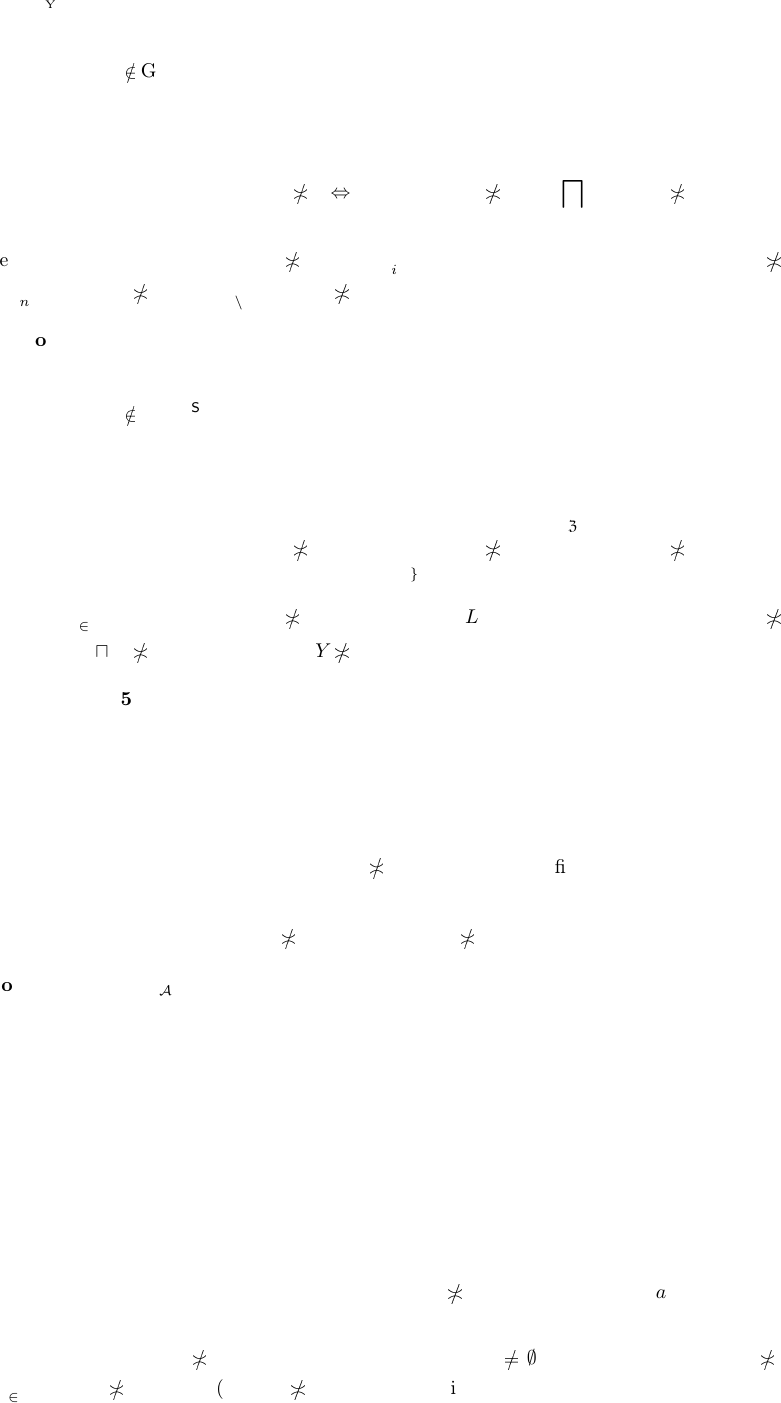Proof. That L
GR ID
A[n]
Strd
if L
k
= 0 for some k n is obvious. It remains to pr ove
L {(k; X Y )} GR ID
A[n]
Strd
L {(k; X)} GR ID
A[n]
Strd
L {(k; Y )} GR ID
A[n]
Strd
.
It is eq uivalent to
l
in\{k}
L
i
(X Y )
A
l
in \{k}
L
i
X
A
l
in \{k}
L
i
Y
A.
Really,
d
in \{k}
L
i
(X Y )
A
d
in \{k}
L
i
X
d
in \{k}
L
i
Y
A
d
in \{k}
L
i
X
A
d
in \{k}
L
i
Y
A.
Proposition 44. Let (A; Z) be a starrish ﬁltrator over a complete meet inﬁnite distributive lattice
and A A. Then id
A[n]
Strd
is a staroid.
Proof. That L
GR id
A[n]
Strd
if L
k
= 0 for some k n is obvious. It remains to prove
L {(k; X Y )} GR id
A[n]
Strd
L {(k; X)} GR id
A[n]
Strd
L {(k; Y )} GR id
A[n]
Strd
.
It is eq uivalent to
l
in\{k}
Z
L
i
(X Y )
A
l
in \{k}
Z
L
i
X
A
l
in \{k}
Z
L
i
Y
A.
Really,
d
in \{k}
Z
L
i
(X Y )
A
d
in \{k}
Z
L
i
X
d
in \{k}
Z
L
i
Y
A
d
in \{k}
Z
L
i
X
A
d
in \{k}
Z
L
i
Y
A.
Proposition 45. Let (A; Z) be a distributive la ttice ﬁltrator with least element and ﬁnitely join-
closed core which is a join semilattice. ID
A[n]
Strd
is a completary staroid for every A A.
Proof. A is a free star by theorem ??4 .47.
L
0
L
i
GR ID
A[n]
Strd
i n: (L
0
L
i
) i A i n: L
0
i L
1
i A i n:
(L
0
i A L
1
i A ) c {0, 1}
n
i n:L
c(i)
i A c {0, 1}
n
:(λi n: L
c(i)
i) GR ID
A[n]
Strd
.
Lemma 46. X GR id
A[n]
Strd
Cor
d
in
A
X
i
A for a join-clo sed ﬁltrator (A; Z) such that both A
and Z are complete lattices, provided that A A.
Proof. X GR id
A[n]
Strd
d
in
Z
X
i
A Cor
d
in
A
X
i
A.
Conjecture 4 7. id
A[n]
Strd
is a completary staroid for every set-th eoretic ﬁlter A.
Proposition 48. Let each (A
i
;Z
i
) for i n (where n is an index set) is a ﬁnitely join-closed ﬁltrator,
such that each A
i
and each Z
i
are join-semilattices. If f is a completary staroid of the form A then
f is a completary staroid of the form Z.
[TODO: Move this proposition (and note its corollary).]
Proof. L
0
Z
L
1
GR f L
0
Z
L
1
GR f L
0
A
L
1
GR f c {0, 1}
n
:
(λi n: L
c(i)
i) GR f c {0, 1}
n
: (λi n: L
c(i)
i) GR f for every L
0
, L
1
Q
Z.
Conjecture 4 9. id
A[n]
Strd
is a completary staroid if A is a ﬁlter o n a set and n is an index set.
6.4 Special case of sets and lters
Proposition 50.
Z
n
X GR id
a[n]
Strd
A a:
Q
X
id
A[n]
for every ﬁlter a on a powerset and
index set n.
Proof. A a:
Q
X
id
A[n]
A a:
T
in
X
i
A
A a:
d
in
P
Z
X
i
A
d
in
P
(
Z
n
X
i
)
a
d
in
P
(
Z
n
X)
i
a
Z
n
X GR id
a[n]
.
Identity staroids and multifuncoids 7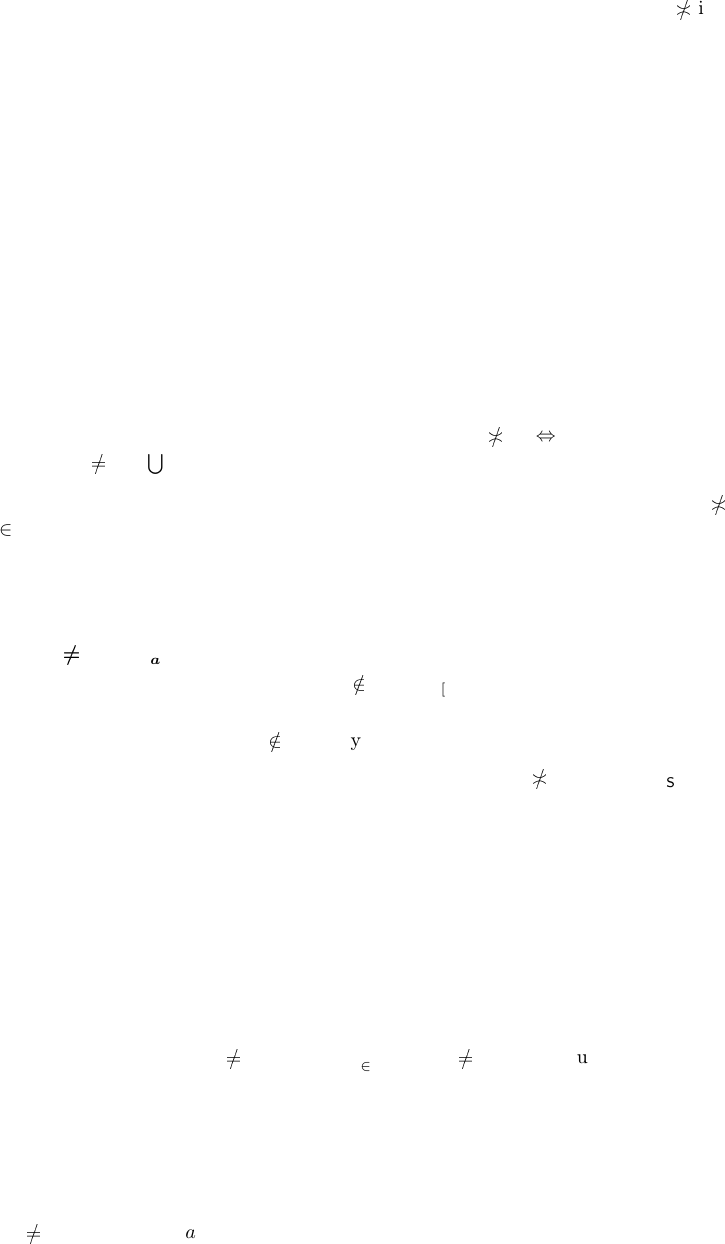Proposition 51. Y GR id
A[n]
Strd
A A: Y GR
Strd
id
A[n]
for every ﬁlter A on a powerset and
Y P
n
.
Proof. Take Y =
Z
n
X.
A A: Y GR
Strd
id
A[n]
A A:
Z
n
X GR
Strd
id
A[n]
A A:
Q
X
id
A[n]
Z
n
X GR id
A[n]
Strd
Y GR id
A[n]
Strd
.
Proposition 52.
Z
n
X GR id
a[n]
Strd
A at Ai n: t X
i
.
Proof.
Z
n
X GR id
a[n]
Strd
A at A: n × {t}
Q
X A at Ai n: t X
i
.
6.5 Relationships between big and small identity staroids
Deﬁnition 53. a
Strd
n
=
Q
in
Strd
a f or every ele ment a of a poset and an index set n.
Proposition 54. id
a[n]
Strd
ID
a[n]
Strd
a
Strd
n
for every ﬁlter a (on any distributive lattice) and an index
set n.
Proof.
GR id
a[n]
Strd
GR ID
a[n]
Strd
. L GR id
a[n]
Strd
up L GR id
a[n]
Strd
L up L: L GR i d
a[n]
Strd
(proposition 4.112??)⇔∀L up LA up a:
d
in
Z
L
i
A L up LA up a:
d
in
Z
L
i
A
0
S
in
L
i
a has ﬁnite intersection property⇔L GR ID
a[n]
Strd
.
GR ID
a[n]
Strd
GR a
Strd
n
. L GR ID
a[n]
Strd
MEET({L
i
| i n} {a}) i a: L
i
a
L GR a
Strd
a
.
Proposition 55. id
a[a]
Strd
ID
a[a]
Strd
= a
Strd
a
for every nontrivial ultraﬁlter a on a se t.
Proof.
GR id
a[a]
Strd
GR ID
a[a]
Strd
. Let L
i
=
Base(a)
i. Then trivially L GR ID
a[a]
Strd
. But to disprove
L GR id
a[a]
Strd
it’s enough to show L
GR id
a[a]
Strd
for some L up L. Really, take
L
i
= L
i
=
Base(a)
i. Then L GR id
a[a]
Strd
A at Ai a: t i what is clearly false (we
can always take i a such that t
i for any point t).
GR ID
a[a]
Strd
= GR a
Strd
a
. L GR ID
a[a]
Strd
i n: L
i
a i a: L
i
a L GR a
Strd
a
.
Corollary 56. a
Strd
a
isn’t an atom when a is a nontrivial ultr aﬁlter.
Corollary 57. Staroidal product of an inﬁnite indexed family of ultraﬁlters may be non-atomic.
Proposition 58. id
a[n]
Strd
is determined by the value of id
a[n]
Strd
. Mo reover id
a[n]
Strd
= id
a[n]
Strd
.
Lemma 59. L GR ID
a[n]
Strd
iﬀ
S
in
L
i
a has ﬁnite intersection property (for primary ltrators).
Proof. LGR ID
a[n]
Strd
d
in
L a
0
F
X
d
in
L a:X
what is equivalent of
S
in
L
i
a
having ﬁnite intersection property.
Proposition 60. ID
a[n]
Strd
is determined by the value of ID
a[n]
Strd
, moreover ID
a[n]
Strd
= ID
a[n]
Strd
(for
primary ﬁltrators).
Proof. L ID
a[n]
Strd
up L ID
a[n]
Strd
up L ID
a[n]
Strd
L up L: L ID
a[n]
Strd
L up L:
d
in
L
i
a
0
F
S
in
L
i
a has ﬁnite intersection property(lemma)⇔L GR ID
a[n]
Strd
.
8 Section 6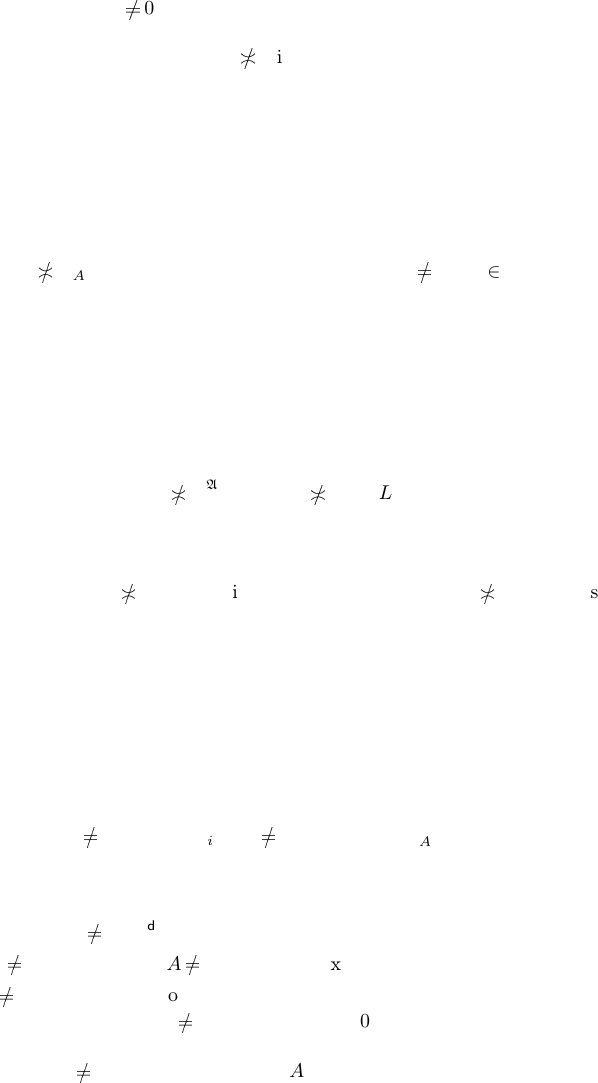Proposition 61. id
a[n]
Strd
ID
a[n]
Strd
for every ﬁlter a and an index set n.
Proof. id
a[n]
Strd
= id
a[n]
Strd
ID
a[n]
Strd
.
Proposition 62. id
a[a]
Strd
ID
a[a]
Strd
for every nontrivial ultraﬁlter a.
Proof. Suppose id
a[a]
Strd
= ID
a[a]
Strd
. Then ID
a[a]
Strd
= ID
a[a]
Strd
= id
a[a]
Strd
above .
Obvious 63. L GR ID
a[n]
Strd
a
d
in
L
i
0
F
if a is an elem e nt of a complete lattice.
Obvious 64. L GR ID
a[n]
Strd
i n: L
i
a i n: L
i
a if a is an ultra ﬁlter on A.
6.6 Identity staroids on principal lters
For principal ﬁlter A (where A is a set) the above deﬁnitions coincide with n-ary identity relation,
as formulated in the below propositions:
Proposition 65.
Strd
id
A[n]
= id
A[n]
Strd
.
Proof. L GR
Strd
id
A[n]
Q
L
id
A[n]
t Ai n: t L
i
T
in
L
i
A
L GR id
A[n]
Strd
.
Thus
Strd
id
A[n]
= id
A[n]
Strd
.
Corollary 66. id
A[n]
Strd
is a principal staroid.
Problem 67. Is ID
A[n]
Strd
principal for every principal ﬁlt er A on a set and index set n?
Proposition 68.
Strd
id
A[n]
ID
A[n]
Strd
for every set A.
Proof. L GR
Strd
id
A[n]
L GR id
A[n]
Strd
A
d
in
A
L
i
A
d
in
Z
L
i
L GR ID
A[n]
Strd
.
Proposition 69.
Strd
id
A[n]
ID
A[n]
Strd
for some set A and index set n.
Proof. L GR
Strd
id
A[n]
d
in
Z
L
i
A what is not implied by
d
in
A
L
i
A that is
L GR ID
A[n]
Strd
. (For a counter example take n = N, L
i
= (0; 1/i), A = R.)
Proposition 70.
Strd
id
A[n]
= id
A[n]
Strd
.
Proof.
Strd
id
A[n]
= id
A[n]
Strd
is obvious from the above.
Proposition 71.
Strd
id
A[n]
ID
A[n]
Strd
.
Proof. X GR
Strd
id
A[n]
up X GR
Strd
id
A[n]
Y up X : Y GR
Strd
id
A[n]
Y up X :
Y id
A[n]
Strd
Y up X :
d
in
Z
Y
i
A
0
d
in
A
X
i
A
0 X GR ID
A[n]
Strd
.
Proposition 72.
Strd
id
A[n]
ID
A[n]
Strd
for some set A.
Proof. We need to prove
Strd
id
A[n]
ID
A[n]
Strd
that is it’s enough to prove (se e the above proof)
that Y up X :
d
in
Z
Y
i
A
0 :
d
in
A
X
i
A
0. A co unter-examp le follows:
Y up X :
d
in
Z
Y
i
A
0 does not hold f or n = N, X
i
= (1/i; 0) for i n, A = (; 0).
To show this, it’s enough to prove
d
in
Z
Y
i
A
0 for Y
i
= (1/i; 0) but this is obvious since
d
in
Z
Y
i
= 0.
On the other hand,
d
in
A
X
i
A
0 for the same X and A.
The above theorems are summarized in the following diagram:
Identity staroids and multifuncoids 9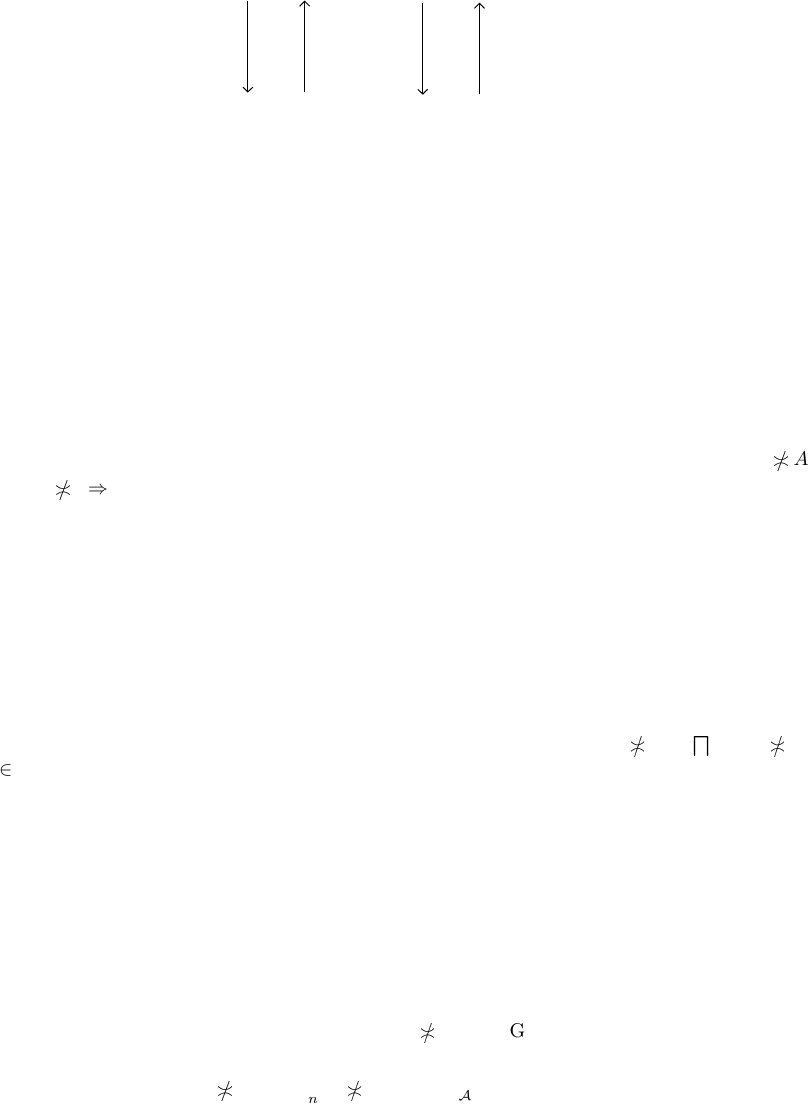Strd
id
A[n]
= id
A[n]
Strd
Strd
id
A[n]
= id
A[n]
Strd
ID
A[n]
Strd
ID
A[n]
Strd
Remark 73. on the diag ram means inequality which can become strict for so me A and n.
6.7 Identity s taroids represented as mee ts and joins
Proposition 74. id
a[n]
Strd
=
d
{↑
Strd
id
A[n]
| A a} for every set- theoretic ﬁlter a where the meet
may be taken on every of the following posets: anchored relations, staroid s.
Proof. That id
a[n]
Strd
Strd
id
A[n]
for every A a is obvious.
Let f
Strd
id
A[n]
for every A a. L GR f L GR
Strd
id
A[n]
A a:
d
in
A
L
i
A
d
in
A
L
i
a L GR id
a[n]
Strd
. Thus f id
a[n]
Strd
.
Proposition 75. ID
A[n]
Strd
=
F
ID
a[n]
Strd
| a atoms A
=
F
{a
Strd
n
| a atoms A} where the meet
may be taken on e very of the following posets: anchored relations, staroids, completary staroids,
provided that A is a ﬁlter on a set.
Proof. ID
A[n]
Strd
ID
a[n]
Strd
for every a atoms A is obvious.
Let f ID
a[n]
Strd
for every a atoms A. Then L GR ID
a[n]
Strd
: L GR f that is
L form f : (MEET({L
i
| i n} {a}) L GR f).
But a atoms A: MEET({L
i
| i n} {a}) a atoms A:
d
in
A
L
i
a
d
in
A
L
i
A
L ID
A[n]
Strd
.
So L ID
A[n]
Strd
L GR f . Thus f ID
A[n]
Strd
.
Then use the fact that ID
a[n]
Strd
= a
Strd
n
.
Proposition 76. id
A[n]
Strd
=
F
id
a[n]
Strd
| a atoms A
where the meet may be taken on every of the
following posets: anchored relations, staroids, provided that A is a ﬁlter on a set.
Proof. id
A[n]
Strd
id
a[n]
Strd
for every a atoms A is obvious.
Let f id
a[n]
Strd
for every a atoms A. Then L GR id
a[n]
Strd
: L GR f that is
L form f :
l
in
Z
L
i
a L GR f
!
.
But a atoms A:
d
in
Z
L
i
a
d
in
Z
L
i
A L id
A[n]
Strd
.
So L id
A[n]
Strd
L GR f. Thus f id
A[n]
Strd
.
7 Finite case
Theorem 77. Let n be a ﬁnite set.
1. id
A[n]
Strd
= ID
A[n]
Strd
if A and Z are meet-semilatt ices and (A; Z) is a ﬁnitely meet-closed ﬁltrator.
10 Section 7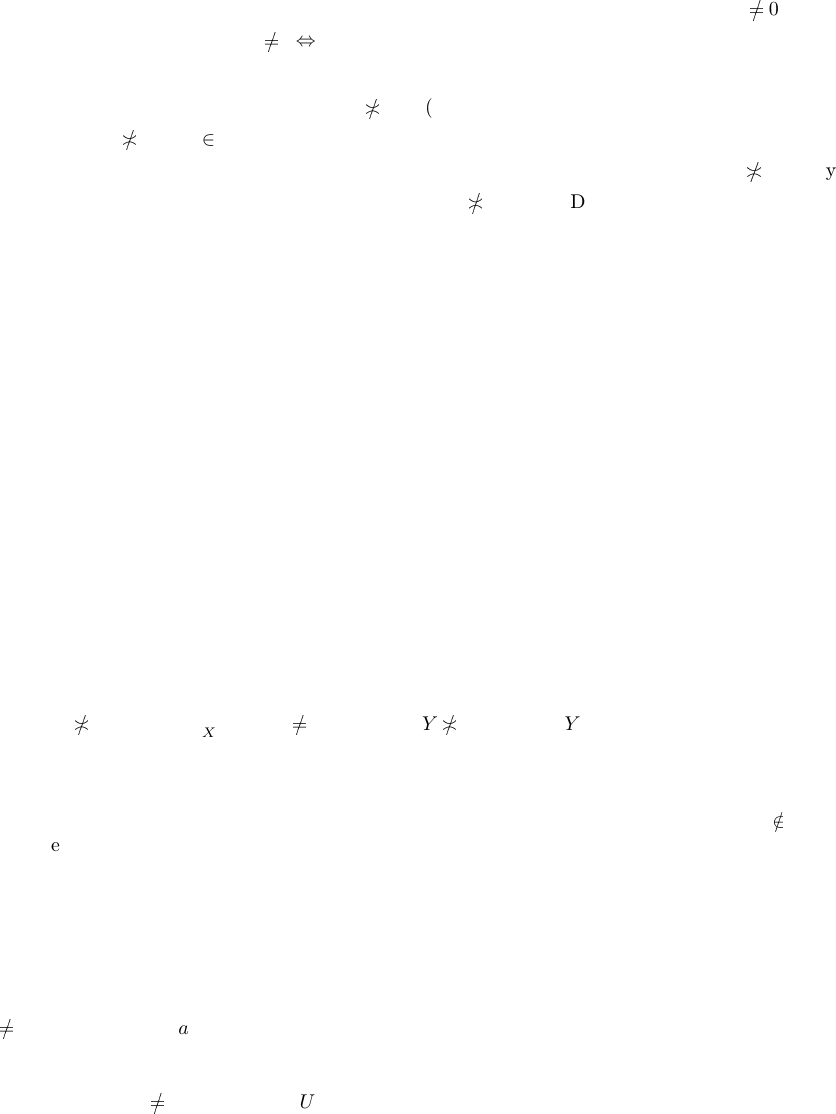2. ID
A[n]
Strd
= id
A[n]
Strd
if (A; Z) is a primary ﬁltrator over a distributive la ttice.
Proof.
1. L GR ID
A[n]
Strd
L GR ID
A[n]
Strd
MEET({L
i
| i n} {A})
d
in
A
L
i
A
0 (by
ﬁniteness)
d
in
Z
L
i
A
0 L id
A[n]
Strd
for every L
Q
Z.
2. L GR id
A[n]
Strd
up L GR id
A[n]
Strd
K up L: K GR id
A[n]
Strd
K up L:
d
in
Z
K
i
A K up L:
d
in
Z
K
i
A (by niteness and theorem 4.44??)⇔∀K up L:
d
in
A
K
i
A A
T
hi
d
in
A
K
i
| K up L
(by the formula for ﬁnite meet of ﬁlters,
theorem 4.111??)⇔A
T
hi
d
in
A
L
i
K
d
in
A
L
i
:A K K
d
in
A
L
i
:A
K (by
separability of core, theorem 4.112??)
d
in
A
L
i
A L ID
A[n]
Strd
.
Proposition 78. Let (A; Z) be a ﬁnitely meet closed ﬁltrator. ID
A[n]
Strd
and id
A[n]
Strd
are the same
for ﬁnite n.
Proof. Because
d
idom L
Z
L
i
=
d
idom L
A
L
i
for nitary L.
8 Counter-examples a nd conjectures
The following example shows tha t the theo rem 33 can’t be stre nghtened:
Example 79. For some multifuncoid f on powersets complete in argument k the following formula
is false:
hf i
l
(L {(k;
F
X)}) =
F
xX
hf i
l
(L {(k ; x)}) for every X P P
k
, L
Q
i(arity f )\{k,l}
F
i
.
Proof. Consider multifuncoid f = Λid
U 
Strd
where U is an inﬁnite set ( of the fo rm P
3
) and L = (Y )
where Y is a nonprincipal lter on U .
hf i
0
(L {(k;
F
X)}) = Y
F
X;
F
xX
hf i
0
(L {(k; x)}) =
F
xX
(Y x).
It can be Y
F
X =
F
xX
(Y x) only if Y is principal: Really: Y
F
X =
F
xX
(Y x)
implies Y
F
X
F
xX
(Y x)
0 x X: Y
x and thus Y is principal. But we claimed
above that it is nonprincipal.
Example 80. There ex ists a staroid f and an indexed family X of princ ipal lters (with arity f =
dom X and (form f)
i
= Base(X
i
) for e very i arity f ), such that f
Q
Strd
X and Y X
GR f
for some Y GR f .
Remark 8 1. Such examples obviously do not exist if both f is a principal staroid and X and Y are
indexed fa milies of principal ﬁlters (be cause for powerset algebras staroidal produ c t is equivale nt
to Cartesian product). This makes the above example inspired.
Proof. (Monroe Eskew) Let a be any (trivial or nontrivial) ultraﬁlter on an inﬁ nite s e t U . Let
A, B a be such that A B A, B. In other words, A, B are arbitrary nonempty sets such that
A B A, B and a be an ultraﬁlter on A B.
Let f be the staroid whose graph consists of functions p: U a such that eit her p(n) A for
all but ﬁnitely many n or p(n) B for all but ﬁnitely many n. Let’s prove f is really a staroid.
It’s obvious px
for every x U . Let k U, L a
U \{k}
. It is enough (taking symmetry into
account) to prove that
L {(k; x y)} GR f L {(k; x)} GR f L {(k; y)} GR f. (1)
Really, L {(k; x y)} GR f iﬀ x y a and L(n) A for all but ﬁnitely many n or L(n) B
for all but ﬁnitely many n ; L {(k; x)} GR f iﬀ x a and L(n) A for all but ﬁnitely many n
or L(n) B; and simila rly for y.
Counter-examples and conjectures 11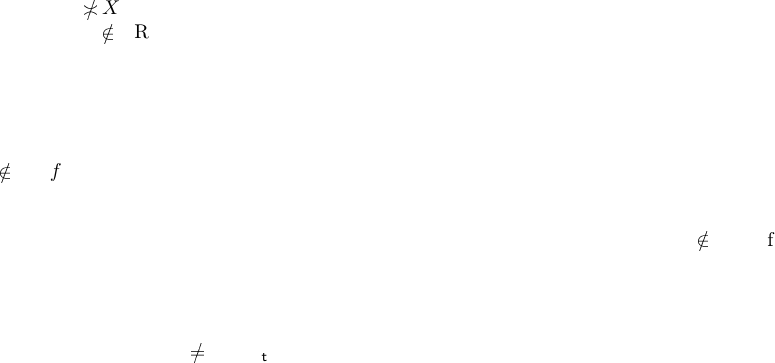But x y a x a y a because a is an ultraﬁlter. So, the formula (1) holds, and we have
proved that f is really a staroid.
Take X be the c onstant function with value A and Y be the constant function with value B.
p GR f : p
X be c ause p
i
X
i
a; so GR f GR
Q
Strd
X that is f
Q
Strd
X.
Finally, Y X
GR f because X Y = λi U: A B.
Some conjectures similar to t he above ex ample:
Conjecture 82. There exists a completa ry staroid f and an indexed family X of principal ﬁl ters
(with arity f = dom X and (form f)
i
= Base(X
i
) for every i arity f), such that f
Q
Strd
X and
Y X
GR f for som e Y GR f .
Conjecture 83. There exists a staroid f and an indexed family x of ultraﬁlters (with arity f =
dom x a nd (form f)
i
= Base(x
i
) for every i arity f), such that f
Q
Strd
x and Y x
GR f for
some Y GR f.
Other conjecture s:
Conjecture 84. If staroid 0
f a
Strd
n
for an ultraﬁlter a and an index set n, then n × {a} G R f .
(Can it be generalized for arbitrary staroidal products?)
Conjecture 8 5. The following posets are atomic:
1. anchored relations on powersets;
2. staroids on powersets;
3. completary star oids on powersets.
Conjecture 8 6. The following posets are atomistic:
1. anchored relations on powersets;
2. staroids on powersets;
3. completary star oids on powersets.
The above conjectures seem diﬃcult, bec ause we know almost not hing about structure of atomic
staroids.
Conjecture 8 7. A staroid on powersets is pri ncipal iﬀ it is com plete in every argument.
Conjecture 8 8. If a is an ultraﬁlter, then id
a[n]
Strd
is an atom of the lattice of:
1. anchored relations of the form ( P Base(a))
n
;
2. staroids of the form (P Base(a))
n
;
3. completary star oids of the form (P Base(a))
n
.
Conjecture 8 9. If a is an ultraﬁlter, then id
a[n]
Strd
is an atom of the lattice of:
1. anchored relations of the form F(Base(a))
n
;
2. staroids of the form F(Base(a))
n
;
3. completary star oids of the form F(Base(a))
n
.
Informal problem: Formulate and prove associativity of staroidal product.
Bib liography
 Victor Porton. Algebraic General Topology. Volume 1. 2014.
12 Section